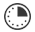# Symmetry Search

JRDAssist
Middle School and High School Teacher

The students will locate manmade objects or things in nature that are symmetrical.

##Length of Time: 1-2 Class Periods

Featured Programs:

### Common Core Alignment

CCSS.Math.Content.4.G.A.3 - Recognize a line of symmetry for a two-dimensional figure as a line across the figure such that the figure can be folded along the line into matching parts. Identify line-symmetric figures and draw lines of symmetry.

## Objectives & Outcomes

The students will be able to define symmetry and identify and list examples of symmetrical objects, both manmade and in nature.

## Materials Needed

drawing paper, pencils/colored pencils or markers, stapler to use

Prepare ahead of time:  Enough sheets of drawing paper of the same size, ¼ or ½ of a regular sheet of paper would be sufficient, supplies for students.  Also, seek locations where students may search for the objects indoors and outdoors.

## Procedure

### Opening to Lesson

• Display a circle, square, and a rectangle with a line through the center.
• Ask students:  What do the three shapes have in common?
• Allow for responses and discussion.  Lead the discussion to the two equal halves of each shape.  They are symmetrical.
• Introduce the term symmetry to the class.

### Body of Lesson

#### Modeling

• Explain to students that many manmade and natural objects are symmetrical.
• Use a couple of symmetrical objects as an example for students.
• Tell students they will be searching for other symmetrical objects throughout the room/school and outdoors.

#### Guided Practice

• Place students in pairs.
• Distribute drawing paper with colored pencils, etc.  The students will also need something to write on when moving outside the room.
• Tell students they are to find as many symmetrical objects as possible, make a sketch of each item, and draw the line of symmetry on each.
• Allow students time inside the classroom, through the school or other indoor location, and outdoors.
• Once completed, the students will organize their drawings in a booklet, stapling the pages together, and creating a cover page with a title.
• Finally, allow each pair of students to show some of their drawings to the class and to tell why they are symmetrical.  If an object is not symmetrical, allow other students to explain why it is not.
• Display completed booklets in an area for other students and/or visitors to review.

#### Independent Practice

• Give students practice in identifying symmetrical shapes using teacher-created, commercial worksheets, or Internet resources.  Students make drawings of objects at home that are symmetrical, share with the class the next day.

### Closing

• Select volunteers to stand in front of the room. Ask if the human body is symmetrical.
• Allow for responses and discussion as to why of why not.

## Assessment & Evaluation

Create a quiz or test with images of objects, and students will identify which are symmetrical.  Students write an explanation for others, a how-to on determining if an object is symmetrical.

## Modification & Differentiation

When drawing objects, tell students to describe in a word what the object is: circular, triangular, square, rectangular, etc.  Students work alone for the symmetry search.  Students vote on the best booklet, drawings, title page, etc.

## Related Lesson Plans

##### Scale It Up

This lesson will allow students to demonstrate knowledge use of scale.

##### Unknown Volumes

Finding the volumes of miscellaneous-sized containers using a known total measurement of one container.  They will do this in as few steps as possible.

##### Numbers By The Book

This lesson will allow students to show a relationship between numbers and a visual representation in a manner that can be used for younger students.

##### Exchanging Time

The lesson is used for students to practice basic time measurement, and understanding the basic units of time.